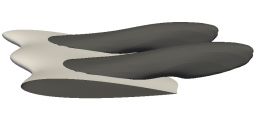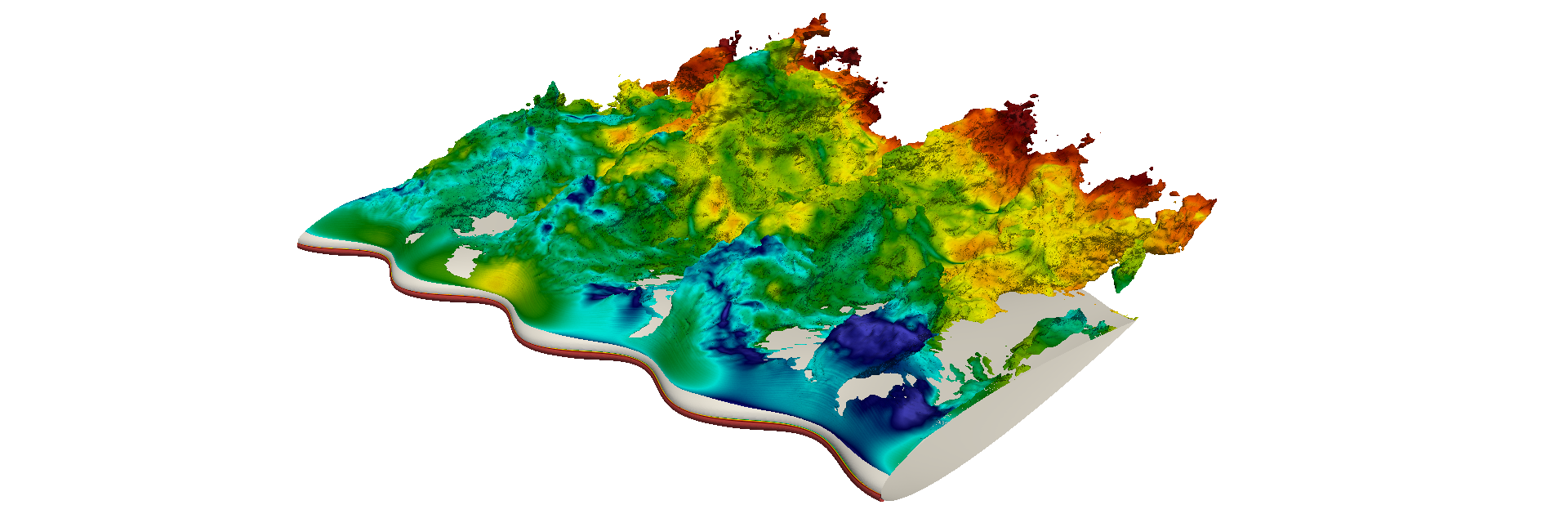# Direct numerical simulations of flow around wavy wings

Nektar++ has been used to investigate the incompressible flow around wings with spanwise waviness, using direct numerical simulations at different Reynolds numbers.

The waviness was imposed by a coordinate transformation, using a novel technique presented in our JCP paper (1). This global mapping allowed us to consider a 2.5D problem, leading to significant advantages in terms of computational cost, and also simplifying the mesh generation procedure.

Results of simulations with Re=1,000 are presented in (2), where we discuss some interesting features of this flow. The following figure shows the recirculation regions (locations where the streamwise velocity is negative) for one of the geometries we considered, with alpha=12 deg, illustrating the distinct separation patterns caused by the waviness.This study was also extended to higher Reynolds numbers. As an example, the following figure shows the recirculation regions coloured by the pressure for a wavy wing with Re=50,000 and alpha=15 deg.